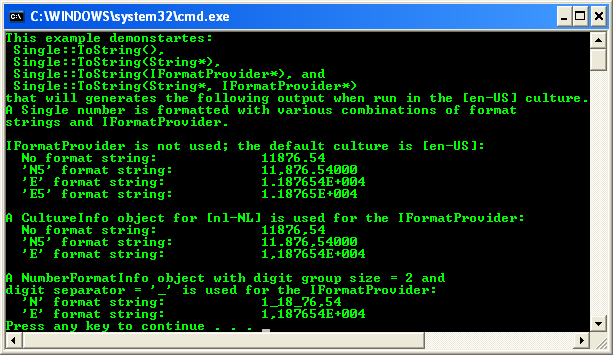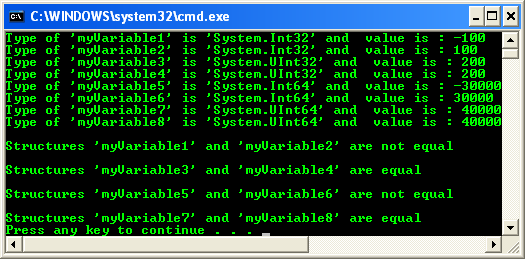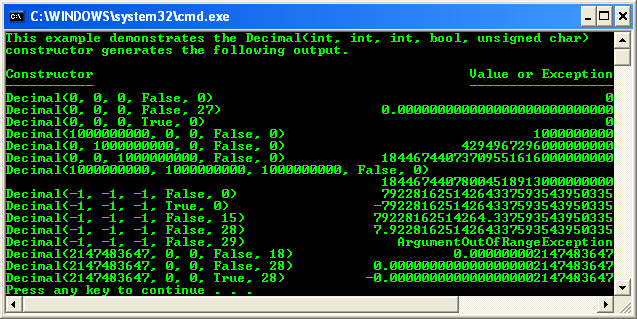# Data Types, Variables and Operators 10

The discussion and the codes used supposed to be based on the new C++ .NET. The following is the topic in this part.

1. # C++ .NET Types Program Examples...continue

 Single structure from System: Single represents a single-precision floating point number. The following code example converts a Single value to a String with the ToString method, using a formatting String. // Var.cpp : main project file. // Example for the Single::ToString() methods.   #include "stdafx.h"   using namespace System; using namespace System::Globalization;   int main(array ^args) {       float singleValue = 11876.54321F;       String^ format = "  {0,-30}{1}";         Console::WriteLine("This example demonstartes:\n"             " Single::ToString(), \n"             " Single::ToString(String*),\n"             " Single::ToString(IFormatProvider*), and \n"             " Single::ToString(String*, IFormatProvider*)\n"             "that will generates the following output when run in the [{0}] "             "culture. \nA Single number is formatted with various "             "combinations of format \nstrings and IFormatProvider.", CultureInfo::CurrentCulture->Name);         // Format the number without and with format strings.       Console::WriteLine("\nIFormatProvider is not "             "used; the default culture is [{0}]:", CultureInfo::CurrentCulture->Name);       Console::WriteLine(format, "No format string:", singleValue.ToString());       Console::WriteLine(format, "'N5' format string:", singleValue.ToString("N5"));       Console::WriteLine(format, "'E' format string:", singleValue.ToString("E"));       Console::WriteLine(format, "'E5' format string:", singleValue.ToString("E5"));         // Create a CultureInfo object for another culture. Use       // [Dutch - The Netherlands] unless the current culture       // is Dutch language. In that case use [English - U.S.].       String^ cultureName = CultureInfo::CurrentCulture->Name->Substring(0, 2)->Equals("nl") ? (String^)"en-US" : "nl-NL";       CultureInfo^ culture = gcnew CultureInfo(cultureName);         // Use the CultureInfo object for an IFormatProvider.       Console::WriteLine("\nA CultureInfo object "             "for [{0}] is used for the IFormatProvider: ", cultureName);       Console::WriteLine(format, "No format string:", singleValue.ToString(culture));       Console::WriteLine(format, "'N5' format string:", singleValue.ToString("N5", culture));       Console::WriteLine(format, "'E' format string:", singleValue.ToString("E", culture));         // Get the NumberFormatInfo object from CultureInfo, and       // then change the digit group size to 2 and the digit       // separator to '_'.       NumberFormatInfo^ numInfo = culture->NumberFormat;       array^sizes = {2};       numInfo->NumberGroupSizes = sizes;       numInfo->NumberGroupSeparator = "_";         // Use a NumberFormatInfo object for IFormatProvider.       Console::WriteLine("\nA NumberFormatInfo object with digit "             "group size = 2 and \ndigit separator = '_' is used "             "for the IFormatProvider:");       Console::WriteLine(format, "'N' format string:", singleValue.ToString("N", culture));       Console::WriteLine(format, "'E' format string:", singleValue.ToString("E", culture));         return 0; }   Output:Note:

1. Int32 structure from System::Int32 represents a 32-bit signed integer.

2. UInt32 structure from System::UInt32 represents a 32-bit unsigned integer.

3. Int64 structure from System::Int64 represents a 64-bit signed integer.

4. UInt64 structure from System::UInt64 represents a 64-bit unsigned integer. This is not a CLS compliant.

The following code example illustrates the use of Equals in the context of Int32, UInt32, Int64 and UInt64, comparing two int values and returning true if they represent the same number, or false if they do not.

// Var.cpp : main project file.

// Illustrates the use of Equals() in the context of Int32, UInt32, Int64, UInt64

// comparing two int values and returning true if they represent

// the same number, or false if they do not.

#include "stdafx.h"

using namespace System;

int main(array<System::String ^> ^args)

{

Int32 myVariable1 = -100;

Int32 myVariable2 = 100;

UInt32 myVariable3 = 200;

UInt32 myVariable4 = 200;

Int64 myVariable5 = -30000;

Int64 myVariable6 = 30000;

UInt64 myVariable7= 40000;

UInt64 myVariable8 = 40000;

// Get and display the declaring type.

Console::WriteLine("Type of 'myVariable1' is '{0}' and  value is : {1}", myVariable1.GetType(), myVariable1);

Console::WriteLine("Type of 'myVariable2' is '{0}' and  value is : {1}", myVariable2.GetType(), myVariable2);

Console::WriteLine("Type of 'myVariable3' is '{0}' and  value is : {1}", myVariable3.GetType(), myVariable3);

Console::WriteLine("Type of 'myVariable4' is '{0}' and  value is : {1}", myVariable4.GetType(), myVariable4);

Console::WriteLine("Type of 'myVariable5' is '{0}' and  value is : {1}", myVariable5.GetType(), myVariable5);

Console::WriteLine("Type of 'myVariable6' is '{0}' and  value is : {1}", myVariable6.GetType(), myVariable6);

Console::WriteLine("Type of 'myVariable7' is '{0}' and  value is : {1}", myVariable7.GetType(), myVariable7);

Console::WriteLine("Type of 'myVariable8' is '{0}' and  value is : {1}", myVariable8.GetType(), myVariable8);

// Compare 'myVariable1' instance with 'myVariable2' Object.

if (myVariable1.Equals(myVariable2))

Console::WriteLine("\nStructures 'myVariable1' and 'myVariable2' are equal");

else

Console::WriteLine("\nStructures 'myVariable1' and 'myVariable2' are not equal");

// Compare 'myVariable3' instance with 'myVariable4' Object.

if (myVariable3.Equals(myVariable4))

Console::WriteLine("\nStructures 'myVariable3' and 'myVariable4' are equal");

else

Console::WriteLine("\nStructures 'myVariable3' and 'myVariable4' are not equal");

// Compare 'myVariable5' instance with 'myVariable6' Object.

if (myVariable5.Equals(myVariable6))

Console::WriteLine("\nStructures 'myVariable5' and 'myVariable6' are equal");

else

Console::WriteLine("\nStructures 'myVariable5' and 'myVariable6' are not equal");

// Compare 'myVariable7' instance with 'myVariable8' Object.

if (myVariable7.Equals(myVariable8))

Console::WriteLine("\nStructures 'myVariable7' and 'myVariable8' are equal");

else

Console::WriteLine("\nStructures 'myVariable7' and 'myVariable8' are not equal");

return 0;

}

Output:Decimal structure from System::Decimal represents a decimal number. The Decimal value type represents decimal numbers ranging from positive 79,228,162,514,264,337,593,543,950,335 to negative 79,228,162,514,264,337,593,543,950,335. The Decimal value type is appropriate for financial calculations requiring large numbers of significant integral and fractional digits and no round-off errors. A decimal number is a floating-point value that consists of a sign, a numeric value where each digit in the value ranges from 0 to 9, and a scaling factor that indicates the position of a floating decimal point that separates the integral and fractional parts of the numeric value. The binary representation of a Decimal value consists of a 1-bit sign, a 96-bit integer number, and a scaling factor used to divide the 96-bit integer and specify what portion of it is a decimal fraction. The scaling factor is implicitly the number 10, raised to an exponent ranging from 0 to 28. Therefore, the binary representation of a Decimal value is of the form, ((-296 to 296) / 10(0 to 28)), where -296-1 is equal to MinValue, and 296-1 is equal to MaxValue.

This type provides methods that convert Decimal values to and from type Char, SByte, Int16, Int32, Int64, Byte, UInt16, UInt32, and UInt64. Conversions from other types to Decimal are widening conversions that never lose information or throw exceptions. Conversions from Decimal to other types are narrowing conversions that round the Decimal value to the nearest integer value toward zero. If the result of the conversion is not representable in the destination type, an OverflowException is thrown. This type provides methods that convert Decimal values to and from Single and Double. Conversions from Decimal to Single or Double are narrowing conversions that might lose precision but not information about the magnitude of the converted value. The conversion will not throw an exception. Conversions from Single or Double to Decimal throw an OverflowException if the result of the conversion is not representable as a Decimal.

The following code example creates several Decimal numbers using the constructor overload that initializes a Decimal structure with three Int32 value words, a Boolean sign, and a Byte scale factor.

// Var.cpp : main project file.

// Example of the Decimal(int, int, int, bool, unsigned char)

// constructor.

#include "stdafx.h"

using namespace System;

// Get the exception type name; remove the namespace prefix.

String^ GetExceptionType(Exception^ ex)

{

String^ exceptionType = ex->GetType()->ToString();

return exceptionType->Substring(exceptionType->LastIndexOf('.') + 1);

}

// Create a Decimal object and display its value.

void CreateDecimal(int low, int mid, int high, bool isNeg, unsigned char scale)

{

// Format the constructor for display.

array<Object^>^boxedParams = gcnew array<Object^>(5);

boxedParams = low;

boxedParams = mid;

boxedParams = high;

boxedParams = isNeg;

boxedParams = scale;

String^ ctor = String::Format("Decimal({0}, {1}, {2}, {3}, {4})", boxedParams);

String^ valOrExc;

// try-catch exception handling block

try

{

// Construct the Decimal value.

Decimal decimalNum = Decimal(low,mid,high,isNeg,scale);

// Format and save the Decimal value.

valOrExc = decimalNum.ToString();

}

catch (Exception^ ex)

{

// Save the exception type if an exception was thrown.

valOrExc = GetExceptionType(ex);

}

// Display the constructor and Decimal value or exception.

int ctorLen = 76 - valOrExc->Length;

// Display the data on one line if it will fit.

if (ctorLen > ctor->Length)

// Otherwise, display the data on two lines.

else

{

Console::WriteLine("{0}", ctor);

Console::WriteLine("{0,76}", valOrExc);

}

}

int main(array<System::String ^> ^args)

{

Console::WriteLine("This example demonstrates the Decimal(int, int, "

"int, bool, unsigned char) \nconstructor "

"generates the following output.\n");

Console::WriteLine("{0,-38}{1,38}", "Constructor", "Value or Exception");

Console::WriteLine("{0,-38}{1,38}", "-----------", "------------------");

// Construct Decimal objects from double values.

CreateDecimal(0, 0, 0, false, 0);

CreateDecimal(0, 0, 0, false, 27);

CreateDecimal(0, 0, 0, true, 0);

CreateDecimal(1000000000, 0, 0, false, 0);

CreateDecimal(0, 1000000000, 0, false, 0);

CreateDecimal(0, 0, 1000000000, false, 0);

CreateDecimal(1000000000, 1000000000, 1000000000, false, 0);

CreateDecimal(-1, -1, -1, false, 0);

CreateDecimal(-1, -1, -1, true, 0);

CreateDecimal(-1, -1, -1, false, 15);

CreateDecimal(-1, -1, -1, false, 28);

CreateDecimal(-1, -1, -1, false, 29);

CreateDecimal(Int32::MaxValue, 0, 0, false, 18);

CreateDecimal(Int32::MaxValue, 0, 0, false, 28);

CreateDecimal(Int32::MaxValue, 0, 0, true, 28);

return 0;

}

Output: